# Forced Convective Heat Transfer - 4 Notes | Study Heat Transfer - Mechanical Engineering

## Mechanical Engineering: Forced Convective Heat Transfer - 4 Notes | Study Heat Transfer - Mechanical Engineering

The document Forced Convective Heat Transfer - 4 Notes | Study Heat Transfer - Mechanical Engineering is a part of the Mechanical Engineering Course Heat Transfer.
All you need of Mechanical Engineering at this link: Mechanical Engineering

4.3.5 Flow across cylinders and spheres

4.3.5.1 Flow across a cylinder
The heat transfer coefficient can be found out by the correlations given by many researchers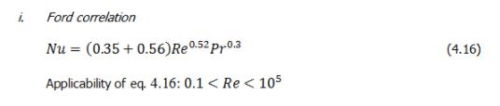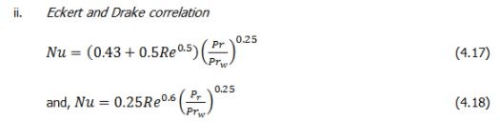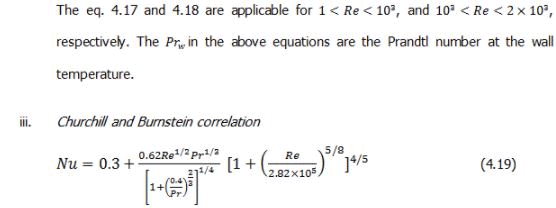Applicability of eq. 4.19: 102 < Re < 107, and Re Pr >0.2 .

However, the following equation (eq. 4.20) is more accurate for the condition where  20,000 < Re< 4,00,000 and Re Pr > 0.2.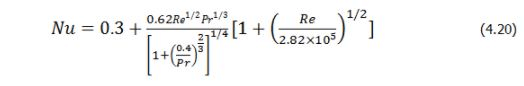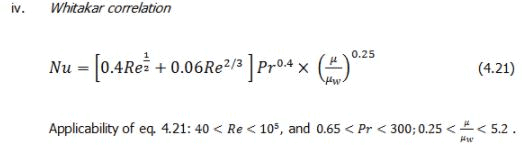4.3.5.2. Flow across a sphere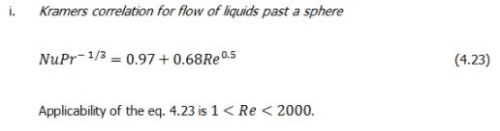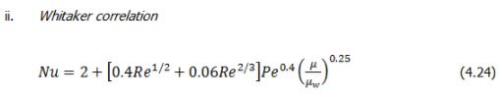The above correlation is applicable to both gases and liquids.

4.3.5.3 Flow over a bank of tubes
Flow over bank of tubes is one of the very important phenomena in chemical process industries. Heat exchanger, air conditioning for cooling and heating etc. involve a bank or bundle of tube over which a fluid flows. The two most common geometric arrangements of a tube bank are shown in fig. 4.5.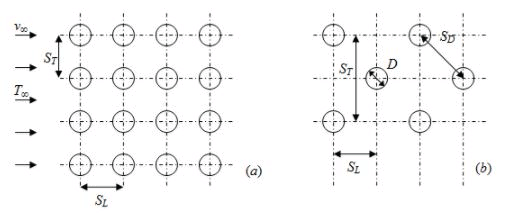Fig.4.5: Tube banks: (a) aligned; (b) staggered

In any of the arrangements, D is the diameter of tube, SL is the longitudinal spacing, and ST is the transverse tube spacing.

The flow over a tube is quite different than the flow over bank of tubes. In case of bank of tube, the flow is influenced by the effects such as the “shading” of one tube by another etc. Moreover, the heat transfer for any particular tube thus not only determined by the incident fluid conditions, ­v and T, but also by D, S, and ST and the tube positions in the bank. It is now clear that the heat transfer coefficient for the first row of tubes is much like that for a single cylinder in cross flow. However, the heat transfer coefficient for the tubes in the inner rows is generally larger because of the wake generation by the previous tubes.

For the heat transfer correlations, in tube banks, the Reynolds number is defined by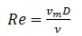where vm is the maximum fluid velocity occurring at the minimum vacant area of the tube bank.
For the aligned tube arrangement,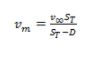(4.25)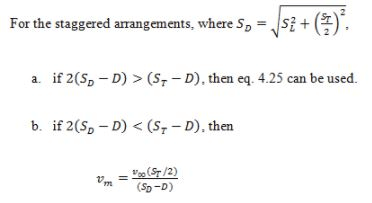(4.26)

In case of bank of tubes, generally we are interested for a single tube but interested to know the average heat transfer coefficient for the entire bank of tubes.
Zukauskas has summarized his extensive for the heat transfer coefficients for fluid past a bank of tubes,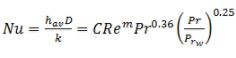(4.27)

The applicability of eq. 4.27: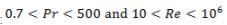, and number of tubes are atleast 20.
The constants C and m of co-relation 5.26 can be found out from any standard book on heat transfer. It may be noted that the above relation is for the inner rows of bank, or for banks of many rows.

The document Forced Convective Heat Transfer - 4 Notes | Study Heat Transfer - Mechanical Engineering is a part of the Mechanical Engineering Course Heat Transfer.
All you need of Mechanical Engineering at this link: Mechanical EngineeringUse Code STAYHOME200 and get INR 200 additional OFF

## Heat Transfer

58 videos|70 docs|85 tests

Track your progress, build streaks, highlight & save important lessons and more!

,

,

,

,

,

,

,

,

,

,

,

,

,

,

,

,

,

,

,

,

,

;